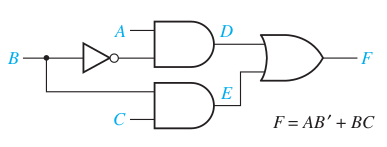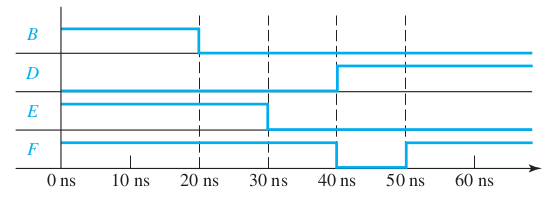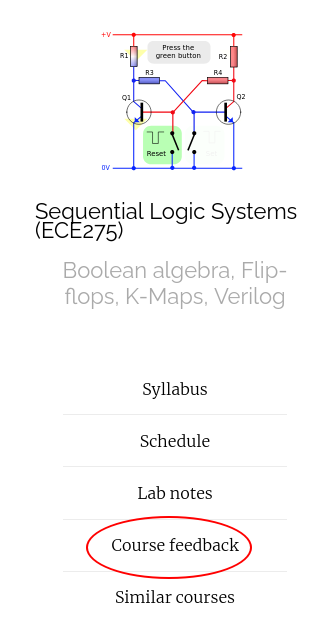## Announcments

• Homework 3 is due on Sept 27th, Monday before class.

## Hazards: Ex1Assume all gates have a propagation delay of 10ns.
1. Identify the transition when static 1 hazard will happen,
2. draw the corresponding timing diagram,
3. and modify the circuit to hazard free circuit.

## Hazards: Ex1 Soln

$f = A\bar{B} + BC$
$$\bar{A}$$ $$A$$
$$\bar{B}$$ $$B$$ $$\bar{B}$$
$$\bar{C}$$ 0 0 0 1
$$C$$ 0 1 1 1
• Identify the variable that is transitioning between them.
• Identify the longer path of the circuit with that variable.
• For a SOP circuit, the transition is that makes to 0->1 for longer circuit and 1->0 for shorter circuit.$$\bar{A}$$ $$A$$
$$\bar{B}$$ $$B$$ $$\bar{B}$$
$$\bar{C}$$ 0 0 0 1
$$C$$ 0 1 1+ 1 1+1

## Hazards: Ex2

Propagation delay of NOT gate=3 ns, AND/OR gate=5 ns

$F = (A + C)(\bA+\bD)(\bB+\bC+D)$## Multi-level synthesis

• SOP and POS are two level circuits
• Fan-in is the number of inputs to a gate
• Two-level circuits have higher fan-in, but smaller propagation delays.
• Fan-in is typically limited by the technology used.

## Approaches Multi-level synthesis

• Factorization
• Functional decomposition

## Factorization Ex1

$f = ABC + ABD + \bar{A}\bar{B}C$

## Factorization Ex2

$f_1 = ABD + CD$ $f_2 = AB\bar{D} + C \bar{D}$

## Functional decomposition Ex1

$f = \bx_1 x_2 x_3 + x_1 \bx_2 x_3 + \bx_1 \bx_2 x_4 + x_1 x_2 x_4$

## Functional decomposition Ex1

$f = \bx_1 x_2 x_3 + x_1 \bx_2 x_3 + \bx_1 \bx_2 x_4 + x_1 x_2 x_4$
$$\bar{x}_1$$ $$x_1$$
$$\bar{x}_2$$ $$x_2$$ $$\bar{x}_2$$
$$\bar{x}_3$$$$\bar{x}_4$$ 0 0 0 0
$$x_4$$ 1 0 1 0
$$x_3$$ 1 1 1 1
$$\bar{x}_4$$ 0 1 0 1

## Functional decomposition Ex2

$f = \sum m(0, 6, 8, 4)$
$$\bar{x}_1$$ $$x_1$$
$$\bar{x}_2$$ $$x_2$$ $$\bar{x}_2$$
$$\bar{x}_3$$$$\bar{x}_4$$ 1 0 0 1
$$x_4$$ 0 0 0 0
$$x_3$$ 0 0 0 0
$$\bar{x}_4$$ 0 1 1 0

## Thanks, Questions, Feedback?

https://vikasdhiman.info/ECE275-Sequential-Logic/